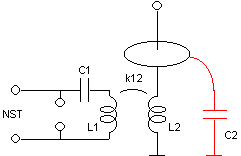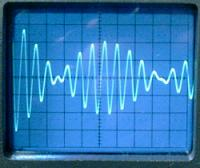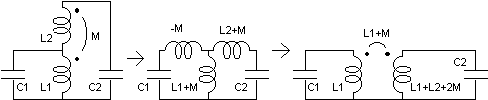A Classical Tesla Coil with Top Load Tuning

(or a 4th-order transformer-coupled multiple resonance network with distributed load capacitance)After several experiments with variants of the Tesla coil circuit, as a transformerless circuit, a capacitively coupled circuit, and a 6th-order directly coupled circuit, I made this more usual version, using a transformer. My main intention was to test the modeling of inductances and mutual inductance, but I also wanted to see how this method improves the performance of these.systems, by allowing independence between the voltage gain and the energy transfer time. The schematic diagram of the system is shown below:The circuit is a classical "Tesla coil", with the only nonusual feature being that tuning is achieved by varying the distributed capacitance of the top terminal, varying the length of the antenna above it. This is the same method that I used in my other systems.

The circuit worked very well, producing streamers and arcs to a grounded object that easily reach 25 cm (specially when the antenna is covered by a half sphere). Its performance is significantly better to what I obtain with a directly coupled system using the same elements, and more regular and insensitive to tuning, certainly due to the faster energy transfer.

C1 (5.07 nF) and L2 (28.2 mH) are the same elements used in my other systems. The neon sign transformer (5 kV, 30 mA) and the spark gap are also the same. L1 is a flat coil, made with insulated #18 solid wire, with 14.7 turns, minimum radius = 7 cm, and maximum radius = 12.5 cm. It was designed to have an inductance of 58.7 µH, and a coupling coefficient with L2 of 0.105. This results in operation in mode 9:10, as my first directly coupled system, but with 5 times bigger input energy.

Design:

This system was designed in the following way: First, the operating mode k:l was selected. This ratio of integers with odd difference (the usual is to have l = k+1) determines the ratio of the two resonance frequencies of the complete system (l/k), and the number of cycles required for complete energy transfer (l/2 cycles of the primary voltage). In this case the mode 9:10 was selected. The coupling coefficient and the element values are obtained from the equations :

L1C1=L2C2=(k2+l2)/(2(w0kl)2)
k12=(l2-k2)/(k2+l2)

From the parameters of L2 (L = 28.2 mH, self-capacitance = 5.55 pF) and C1 (C = 5.07 nF), and adding 5 pF, to account for the top terminal and antenna, to the total capacitance C2 (C = 10.55 pF), L1 is obtained as 58.7 µH, and w0/(2p), the base frequency that multiplies k and l to produce the two resonances, as 30.84 kHz. The two resonances are then ideally at 277.58 kHz and 308.43 kHz. The separate pairs L1-C1 and L2-C2 resonate at 291.79 kHz. The required coupling coefficient is k12 = 0.105.

The secondary coil has 32 cm of length, 4.4 cm of radius, and 1152 turns of #32 wire. The primary coil dimensions can be calculated from this version of Wheeler's formula for flat spirals:

L = 100/2.54 ((r1+r2)N)2/(60r2-28 r1) µH

where r1 is the internal radius, r2 the external radius, both in meters, and N is the number of turns. Choosing r1 = 0.07 m and r2 = 0.125 m, 14.7 turns are required. The coupling coefficient was calculated numerically by Neumann's formula, implemented in my Teslasim program. In this case the required coupling is obtained with L1 2.7 cm below L2. Since I mount L2 in a support that rises it by 3 cm, I mounted L1 over a plastic disk with a hole for the base of L2 at the center, "sewing" the wire coil over the disk with silicone string. In this way L1 would stay at approximately the correct distance, and it would be easy to adjust the distance by rising L1 or L2.Measurements in the assembled system, after proper tuning at low power, showed that L1 ended a bit larger than calculated, with 59.8 µH, and that the coupling was also increased, to ~0.12. The resonances were measured as 289 kHz for C1-L1, and 270 kHz - 305 kHz for the whole system. The observed waveforms put the operating mode between 7:8 and 8:9. The losses caused by the tuner in the primary circuit cause some distortion in the ideal waveform, explaining the apparent inconsistency between the number of cycles before the first notch and until the second notch. A simulation including 3 ohms of resistance in the primary circuit agrees well with the observation. The increased primary inductance can be atributed to wiring inductances in the setup (it would be enough the complete the 15th turn to exceed the measured inductance). The increased coupling may be due to wiring, due to capacitive coupling (note that my capacitive transformer system has a similar structure, but in this case the capacitance between L2 and L1 is very small), or, more probably, due to the nonuniform current distribution in L2, and even due to the presence of a shorted turn caused by the top terminal, not considered in the calculations. It was easy to adjust the system for correct operation in modes 7:8 or 9:10, by moving L1 by less than1 cm. Actually, the difference in efficiency between these modes or anywhere between them is insignificant.An interesting observation is that the effective coupling can be substantially changed if L2 is connected to one side of L1, with the other side of L1 grounded. If I connect L2 to the center of L1 and ground its outer side, the mode decreases to close to mode 6:7, and there is a visible improvement in the performance. Reversing the connections of L1 is also possible, but it decreases the effective coupling. The observed decrease was to mode 12:13. This connection is knows as "Oudin coil", and was first described in 1892. In this case, it was made with two separate coils.

An Oudin coil with inductances L1 and L2, and coupling coefficient M, can be transformed into an equivalent Tesla coil by the aplication of the "T" equivalent of a transformer twice, as shown below:The result is a Tesla coil with the parameters:

L1'=L1
L2'=L1+L2+2M
M'=L1+M
k12'=(L1+M)/(L1(L1+L2+2M))1/2

In the example, using the observed k12 = 0.12, k12' = 0.16, corresponding closely to a bit less than the observed mode 6:7. The reverse connection corresponds to a negative M, what gives k12' = 0.075, corresponding to a bit more than the observed mode 12:13.

Programs that can design and simulate the behavior of this system, and others, can be found here. Extensive materials about Tesla coils can be found in the archives of the Tesla list.

A short video with a demonstration of the device in operation in March 2006.

 See the papers about "multiple resonance networks" here.

Warning:

This device is powered by a power source that has enough voltage, and specially enough current, to give a fatal shock. The NST, the terminals of C1, L1, and the spark gap must not be touched in any circunstance while the system is energized. The high-voltage arcs are also not safe to touch. They may cause burns, and the current is intense enough to cause internal body damage.

Created: 28 February 2003
Last update: 5 April 2006
Created and maintained by Antonio Carlos M. de Queiroz